# How Machine Learning Leverages Linear Algebra to Solve Data Problems

Why you should learn the fundamentals of linear algebra.Source: https://www.wiplane.com/p/foundations-for-data-science-ml

Machines or your computers only understand numbers and these numbers need to be represented and processed in a way that enables these machines to solve problems by learning from data instead of predefined instruction as in the case of programming.

All types of programming use mathematics at some level and machine learning is programming data to learn the function that best describes the data.

The problem(or process) of finding the best parameters of a function using data is called model training in ML.

Therefore, in a nutshell, machine learning is programming to optimize for the best possible solution and we need math to understand how that problem is solved.

The first step towards learning Math for ML is Linear algebra.

Linear Algebra is that mathematical foundation that solves the problem of representing data as well as computations in machine learning models.

It is the math of arrays — technically referred to as vectors, matrices, and tensors.

## Common Areas of Application — Linear Algebra in Action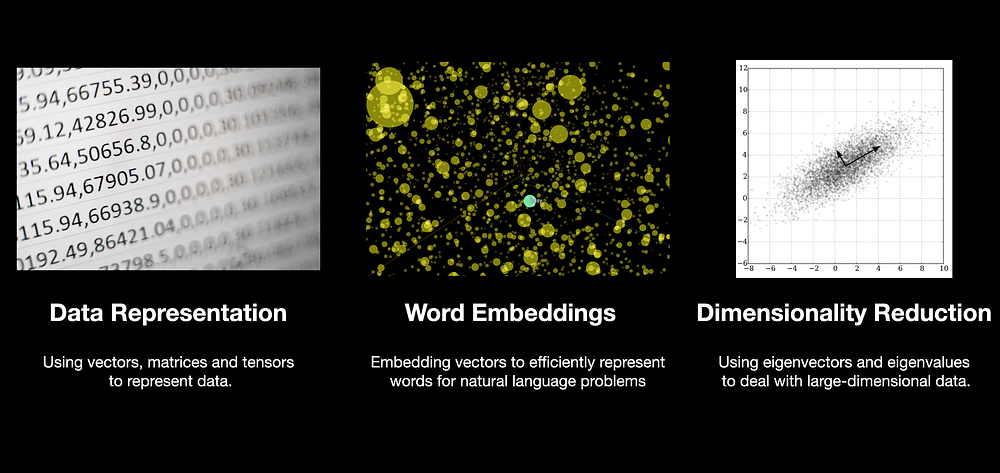Source: https://www.wiplane.com/p/foundations-for-data-science-ml

In the ML context, all major phases of developing a model have linear algebra running behind the scenes.

Important areas of application that are enabled by linear algebra are:

• data & learned model representation
• word embeddings
• dimensionality reduction

Data Representation — The fuel of ML models, a.k.a. data, needs to be converted into arrays before feeding into your models, the computations performed on these arrays include operations like matrix multiplication(dot product) which further returns the output that is also represented as a transformed matrix/tensor of numbers.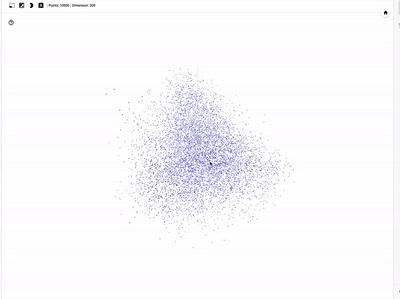https://projector.tensorflow.org/

Word embeddings — don’t worry about the terminology here, it is just about representing large-dimensional data(think of a huge number of variables in your data) with a smaller dimensional vector.

Natural Language Processing(NLP) deals with textual data. Dealing with text means comprehending the meaning of a large corpus of words and each word represents a different meaning which might be similar to another word, vector embeddings in linear algebra allow us to represent these words more efficiently.

Eigenvectors(SVD) — Finally, concepts like eigenvectors allow us to reduce the number of features or dimensions of the data while keeping the essence of all of them using something called principal component analysis.

## From data to Vectors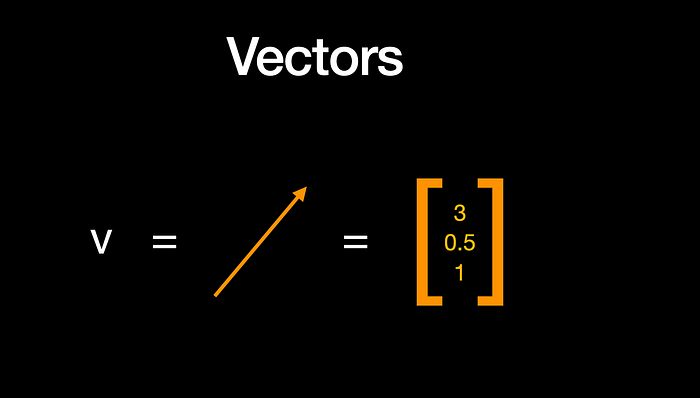Source: https://www.wiplane.com/p/foundations-for-data-science-ml

Linear algebra basically deals with vectors & matrices(different shapes of arrays) and operations on these arrays. In NumPy, vectors are basically a 1-Dimensional array of numbers but geometrically, this has both magnitude and direction.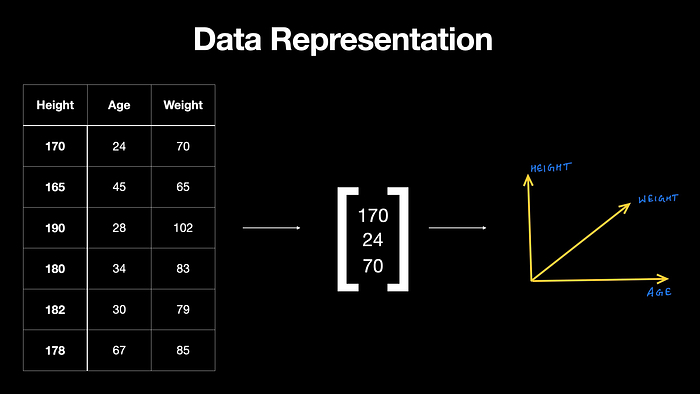Source: https://www.wiplane.com/p/foundations-for-data-science-ml

Our data can be represented using a vector. In the figure above, one row in this data is represented by a feature vector that has 3 elements or components representing 3 different dimensions. N-entries in a vector make it n-dimensional vector space and in this case, we can see 3-dimensions.

## Deep Learning — Tensors flowing through a neural network

Linear algebra can be seen in action across all the major applications today, be it sentiment analysis on a LinkedIn or a Twitter post(embeddings), be it detecting a type of lung infection from X-ray images(computer vision), or any speech to text bot(NLP).

All of these data types are represented by numbers in tensors and we run vectorized operations to learn patterns from them using a neural network which then outputs processed tensor which in turn is decoded to produce the final inference of the model.

## Dimensionality Reduction — Vector space transformation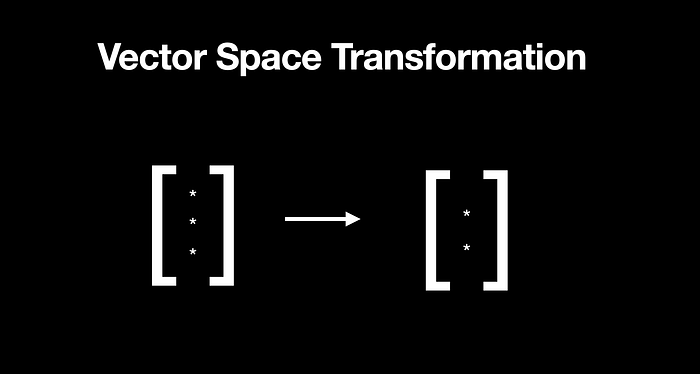Source: https://www.wiplane.com/p/foundations-for-data-science-ml

When it comes to embeddings, you can basically think of an n-dimensional vector being replaced with another vector that belongs to a lower-dimensional space which is more meaningful and the one that overcomes computational complexities.

For example, here is a 3-dimensional vector that is replaced by a 2-dimensional space but you can extrapolate it to a real-world scenario where you have a very large number of dimensions.

Reducing dimensions doesn’t mean dropping features from the data but finding new features that are linear functions of the original features and preserves the variance of the original features.

Finding these new variables(features) translates to finding the principal components(PCs) which converge to solving eigenvectors and eigenvalues problems.

## Recommendation Engines — Making use of embeddings

You can think of Embedding as a 2D plane being embedded in a 3D space and that’s where this term comes from. You can think of the ground you are standing on as a 2D plane that is embedded into this space in which we live.

Just to give you a real-world use case to relate to all of this discussion on vector embeddings, all applications that are giving you personalized recommendations are using vector embedding in some form.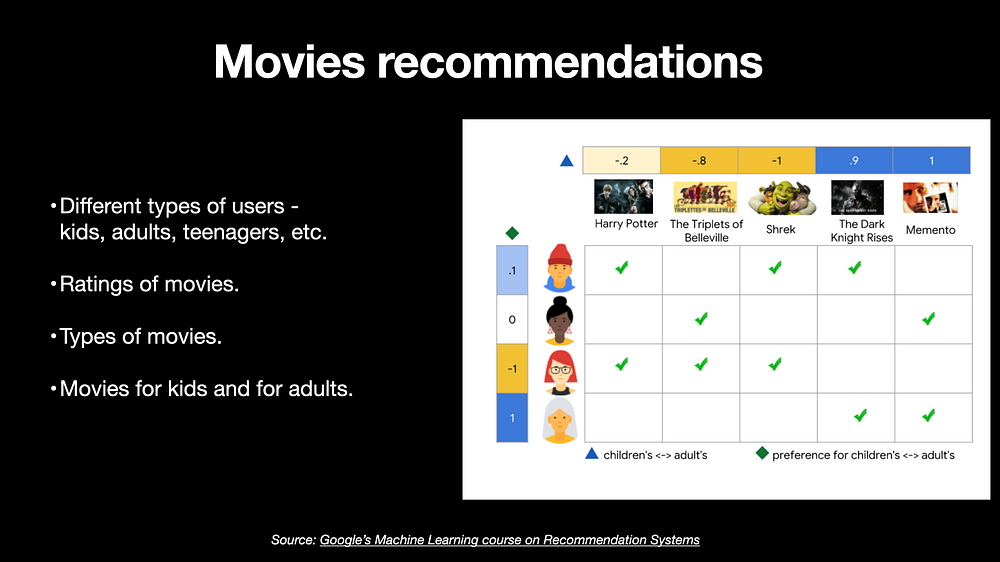For example, here is a graphic from Google’s course on recommendation systems where we are given this data on different users and their preferred movies. Some users are kids and others are adults, some movies were are all-time classics while others are more artistic. Some movies are targeted towards a younger audience while movies like memento are preferred by adults.

Now, we not only need to represent this information in numbers but also need to find new smaller dimensional vector representations that capture all these features well.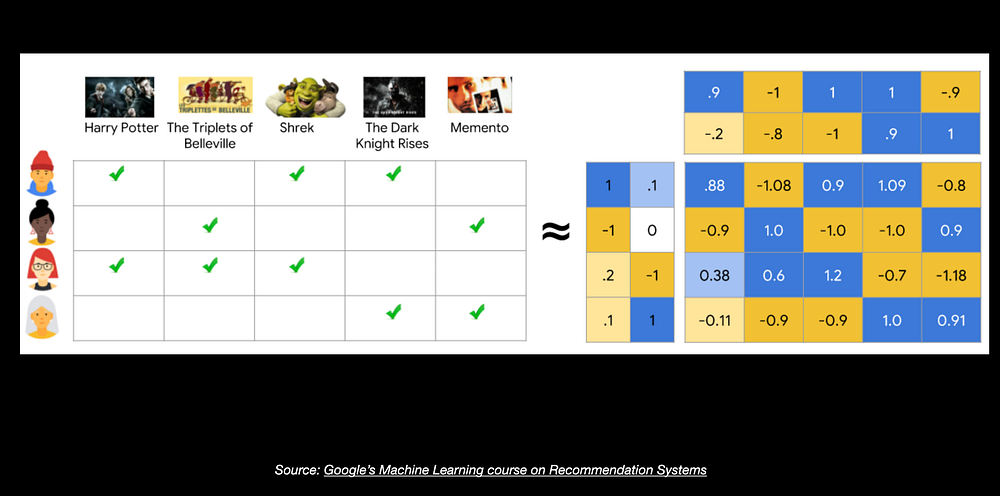Source: Google’s Machine Learning Course on recommendation systems

A very quick way to understand how we can pull this task is by understanding something called Matrix Factorization which allows us to break a large matrix down into smaller matrices.

Ignore the numbers and colors for now and just try to understand how we have broken down one big matrix into two smaller ones.

For example, here this matrix of 4X5, 4 rows, and 5 features were broken down into two matrices, one of the shape 4X2 and the other of shape 2X5. We basically have new smaller dimensional vectors for users and movies.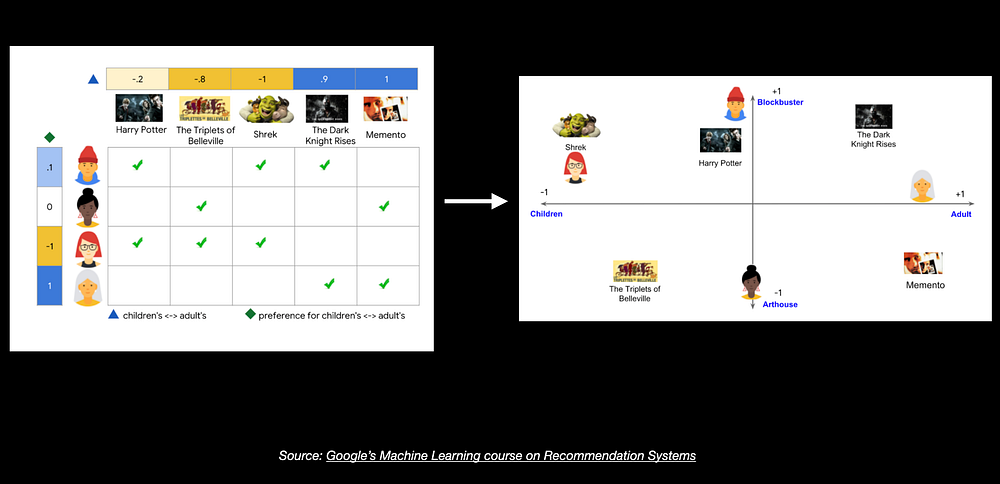And this allows us to plot this on a 2D vector space, and here you’ll see that user #1 and the movie Harry Potter are closer and user #3 and the movie Shrek are closer.

The concept of dot product(matrix multiplication) of vectors tells us more about the similarity of two vectors. And it has applications in correlation/covariance calculation, linear regression, logistic regression, PCA, convolutions, PageRank, and numerous other algorithms.

## Industries where you see LA being used heavily

By now, I hope you are convinced that Linear algebra is driving the ML initiatives in a host of areas today. If not, here is a list to name a few:

• Statistics
• Chemical Physics
• Genomics
• Word Embeddings — neural networks/deep learning
• Robotics
• Image Processing
• Quantum Physics

## How much should you know to get started with ML / DL

Now, the important question is how can you learn to program these concepts of linear algebra. So, the answer is you don’t have to reinvent the wheel, you just need to understand the basics of vector algebra computationally and you then learn to program those concepts using NumPy.

NumPy is a scientific computation package that gives us access to all the underlying concepts of linear algebra. It is fast as it runs compiled C code and it has a large number of mathematical and scientific functions that we can use.

## Recommended resources

👉 You can use the code `TDS10` to get 10% off.

Check out the course outline:

Bio: Harshit Tyagi is an engineer with amalgamated experience in web technologies and data science(aka full-stack data science). He has mentored over 1000 AI/Web/Data Science aspirants, and is designing data science and ML engineering learning tracks. Previously, Harshit developed data processing algorithms with research scientists at Yale, MIT, and UCLA.

Original. Reposted with permission.

Related: